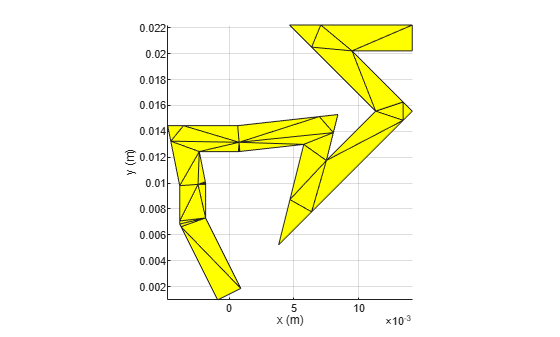# tracePoint

Create custom line trace based on specified X and Y coordinates

## Description

Use the `tracePoint` object to create a custom line trace by tracing a line along the specified X and Y coordinates.

## Creation

### Syntax

``trace = tracePoint``
``trace = tracePoint(Name=Value)``

### Description

example

````trace = tracePoint` creates a line trace using default properties.```

example

````trace = tracePoint(Name=Value)` sets Properties using one or more name-value arguments. For example, `tracePoint(Width=0.0050)` creates a line trace with the width of `0.0050`. Properties not specified retain their default values.```

## Properties

expand all

Name of the custom line trace, specified as a character vector or string scalar.

Example: `trace = tracePoint(Name='tracepoint1')`

Data Types: `char` | `string`

Coordinates of custom line trace, specified as a n-by-2-array of X and Y coordinates.

Example: `trace = tracePoint(TracePoints=[0 0;0 -10;6 -10;6 0]])`

Data Types: `double`

Width of the line trace, specified as a positive scalar in meters. This value is applied to all line segments in the custom trace.

Example: `trace = tracePoint(Width=0.005)`

Data Types: `double`

Corner where two line segments interface, specified as either `"Sharp"`, `"Miter"`, or `"Smooth"`. To apply the same value to all corners, specify a string scalar. For a different value for all corners, specify a (n-2)-by-1 vector of strings.

Example: `trace = tracePoint(Corner="Miter")`

Data Types: `string`

## Object Functions

 `add` Boolean unite operation on two RF PCB shapes `subtract` Boolean subtraction operation on two RF PCB shapes `intersect` Boolean intersection operation on two RF PCB shapes `plus` Shape1 + Shape2 for RF PCB shapes `minus` Shape1 - Shape2 for RF PCB shapes `and` Shape1 & Shape2 for RF PCB shapes `area` Calculate area of RF PCB shape in square meters `rotate` Rotate RF PCB shape about defined axis `rotateX` Rotate RF PCB shape about x-axis `rotateY` Rotate RF PCB shape about y-axis and angle `rotateZ` Rotate RF PCB shape about z-axis `translate` Move RF PCB shape to new location `scale` Change size of RF PCB shape by fixed amount

## Examples

collapse all

Create a custom line using default properties.

`customLine = tracePoint`
```customLine = tracePoint with properties: Name: 'mytracePoint' TracePoints: [10×2 double] Width: 0.0020 Corner: "Sharp" ```

View the trace.

`show(customLine)`Create a custom line trace using default properties.

`customLine = tracePoint;`

Rotate the trace by 45 degrees along the Z-axis.

`customLine = rotateZ(customLine,45)`
```customLine = tracePoint with properties: Name: 'mytracePoint' TracePoints: [10×2 double] Width: 0.0020 Corner: "Sharp" ```

View the trace.

`show(customLine)`Mesh the custom line trace at a maximum edge length of 1 m.

`mesh(customLine,MaxEdgeLength=1)`Create a custom trace line with smooth corners.

`customLine = tracePoint(Name='tracepoint',Corner="Smooth")`
```customLine = tracePoint with properties: Name: 'tracepoint' TracePoints: [10x2 double] Width: 0.0020 Corner: "Smooth" ```
`show(customLine)`## Version History

Introduced in R2021b